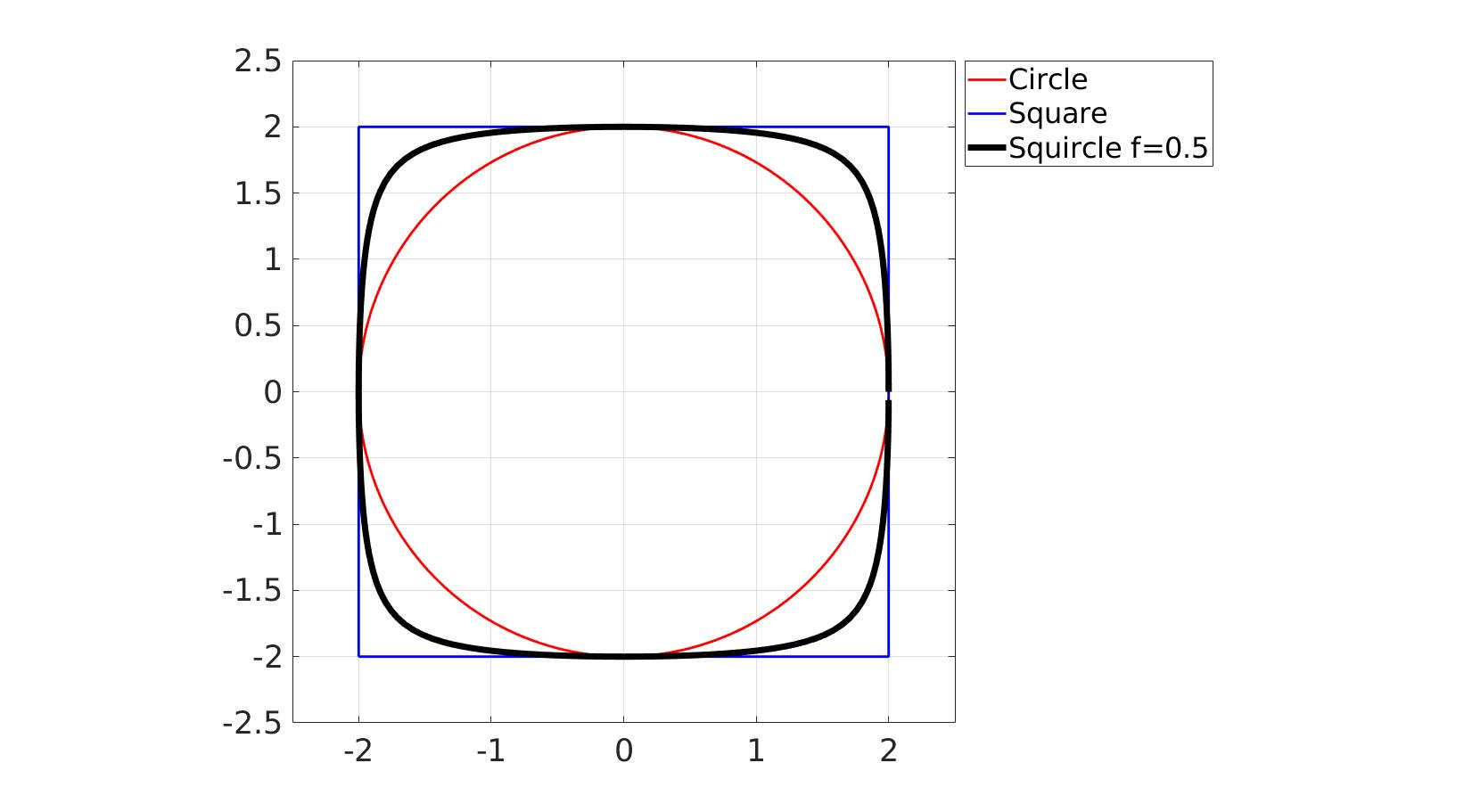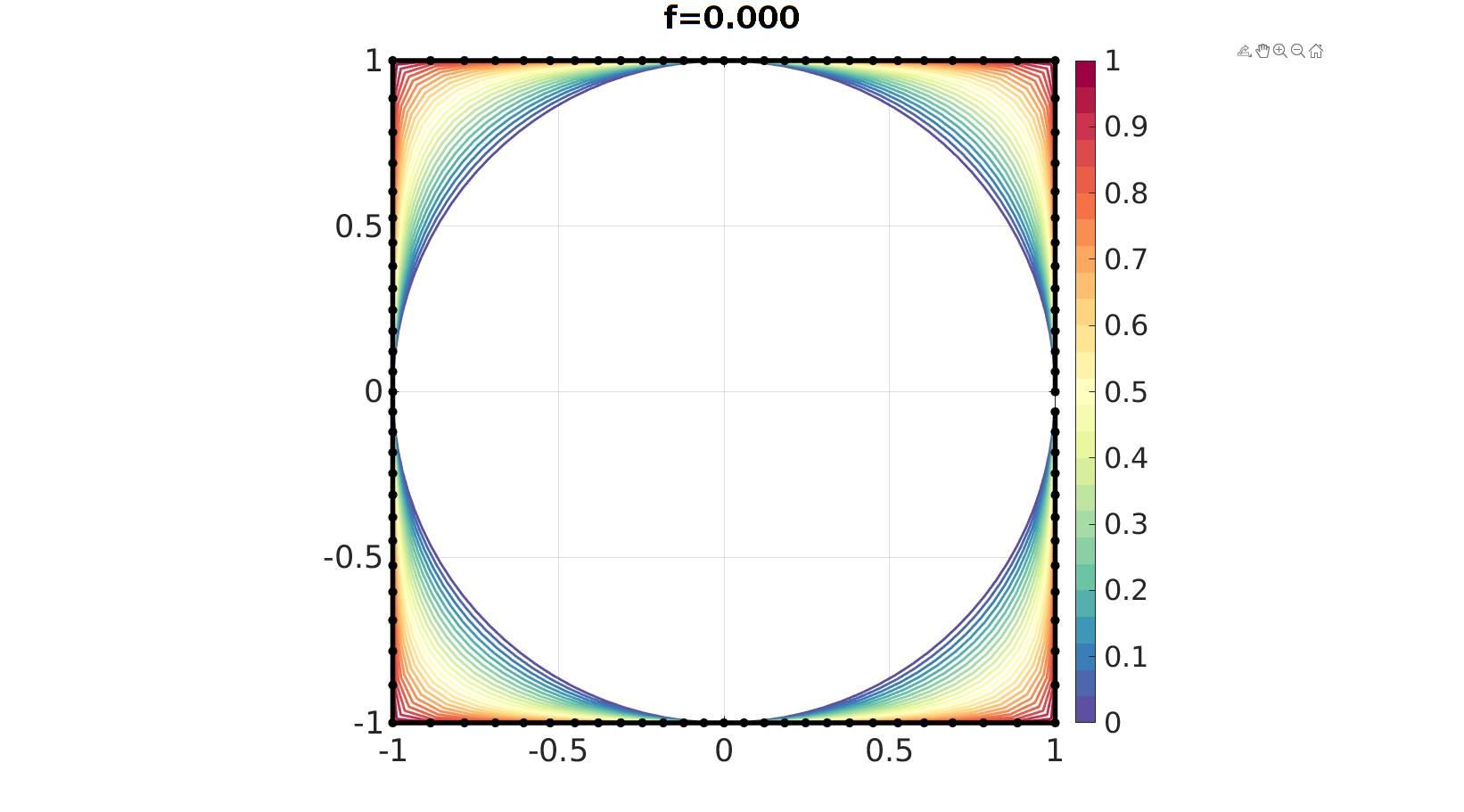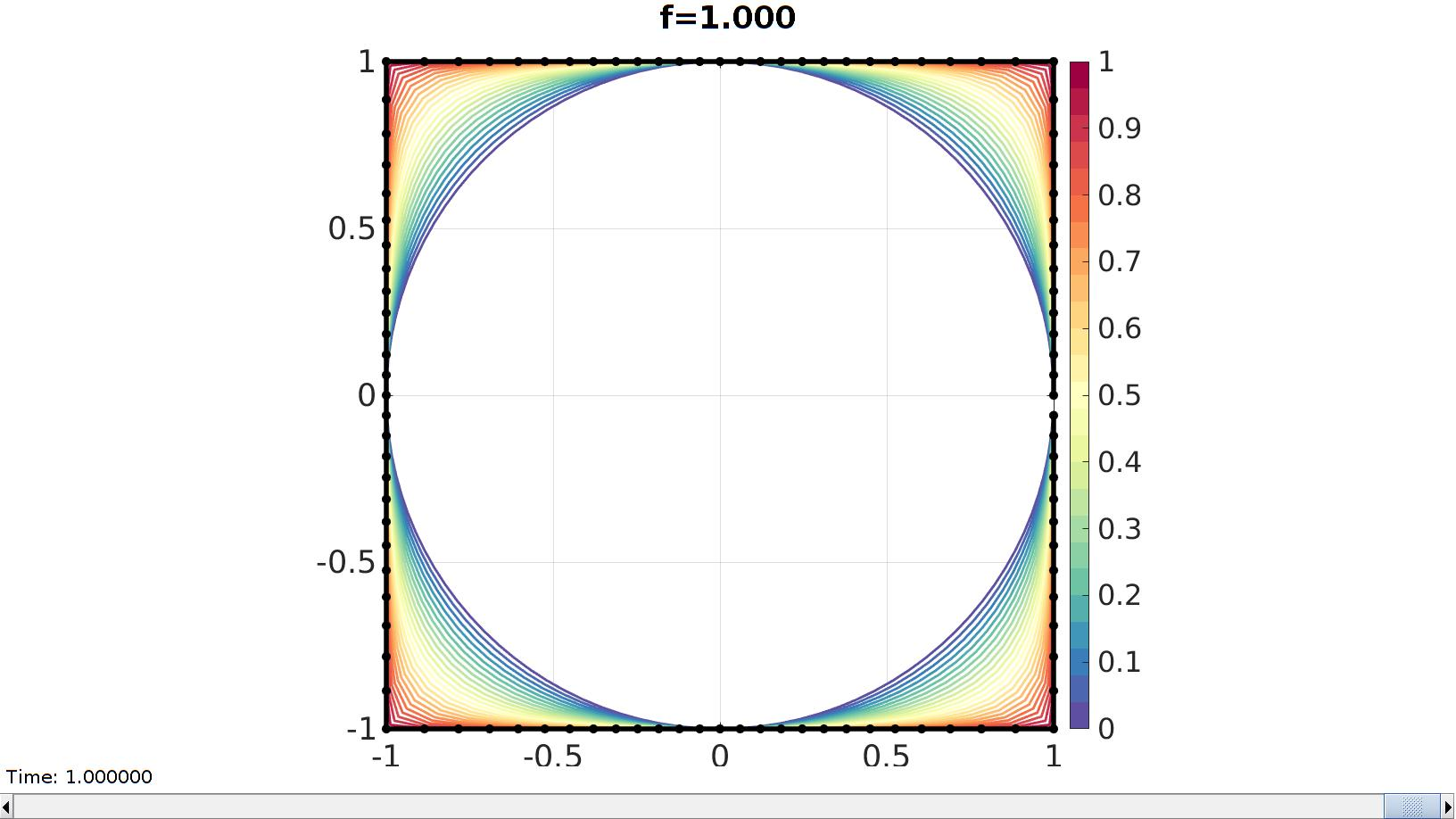# squircle

Below is a demonstration of the features of the squircle function

## Contents

```clear; close all; clc;
```

## Syntax

[V]=squircle(r,f,n);

## Description

The squircle is a shape intermediate between a circle and a square. This function outputs the coordinates V for a squircle using the input radius r, the weighting parameter f, and the number of points n.

This implementation is based on: https://arxiv.org/vc/arxiv/papers/1604/1604.02174v1.pdf

## Example 1: Constructing a squircle

```n=200; %Number of points on curve
```
```f=0.5;
[V]=squircle(r,f,n);
```
```t=linspace(0,2*pi,n)';
Vc=r*[cos(t) sin(t) zeros(size(t))]; %Circle coordinates
Vs=r*[-1 -1 0; 1 -1 0; 1 1 0; -1 1 0; -1 -1 0];
```

Visualization

```cFigure; hold on
hp1=plotV(Vc,'r-' ,'LineWidth',2);
hp2=plotV(Vs,'b-' ,'LineWidth',2);
hp3=plotV(V ,'k.-','LineWidth',5);

axis equal; axis tight; grid on; box on;
set(gca,'FontSize',25);
axis(r*[-1.25 1.25 -1.25 1.25]);
legend([hp1 hp2 hp3],{'Circle','Square',['Squircle ','f=',num2str(f)]},'Location','NorthEastOutSide')
drawnow;
```## Example 2: Animate effect of varying f

```n=104; %Number of points on curve

nSteps=25; %Number of animation steps
f=linspace(0,1,nSteps);
```
```hf=cFigure; hold on
ht=gtitle(['f=',sprintf('%.3f',f(1))],25);
c=spectral(nSteps);
for q=1:1:nSteps
[V]=squircle(r,f(q),n);
hp1=plotV(V,'k-','LineWidth',2);
hp1.Color=c(q,:);
end

hp2=plotV(V,'k.-','LineWidth',4,'MarkerSize',25);
axis equal; axis tight; grid on; box on;
colormap(c); colorbar; caxis([0 1]);
set(gca,'FontSize',25);
drawnow;
``````%Populate the animation structure

%Create the time vector
animStruct.Time=linspace(0,1,nSteps);

for q=1:1:nSteps
[V]=squircle(r,f(q),n);

%Set entries in animation structure
animStruct.Handles{q}=[hp2 hp2 ht]; %Handles of objects to animate
animStruct.Props{q}={'XData','YData','String'}; %Properties of objects to animate
animStruct.Set{q}={V(:,1),V(:,2),['f=',sprintf('%.3f',f(q))]}; %Property values for to set in order to animate
end

anim8(hf,animStruct);
```GIBBON www.gibboncode.org

Kevin Mattheus Moerman, [email protected]

GIBBON footer text

GIBBON: The Geometry and Image-based Bioengineering add-On. A toolbox for image segmentation, image-based modeling, meshing, and finite element analysis.

Copyright (C) 2006-2021 Kevin Mattheus Moerman and the GIBBON contributors

This program is free software: you can redistribute it and/or modify it under the terms of the GNU General Public License as published by the Free Software Foundation, either version 3 of the License, or (at your option) any later version.

This program is distributed in the hope that it will be useful, but WITHOUT ANY WARRANTY; without even the implied warranty of MERCHANTABILITY or FITNESS FOR A PARTICULAR PURPOSE. See the GNU General Public License for more details.

You should have received a copy of the GNU General Public License along with this program. If not, see http://www.gnu.org/licenses/.